# RRB ALP 2018 Practice Test Papers | Arithmetic Questions (Day-78)

Dear Aspirants, Here we have given the Important RRB ALP & Technicians Exam 2018 Practice Test Papers. Candidates those who are preparing for RRB ALP 2018 can practice these Arithmetic Questions to get more confidence to Crack RRB 2018 Examination.

[WpProQuiz 3096]

Click “Start Quiz” to attend these Questions and view Solutions

1) Find the missing term

3, 46, 92, 161, 253, 368, x

a) 500

b) 502

c) 504

d) 506

2)  In 10 days a project was completed by 12 workers. If the work is to be completed 2 days before the scheduled time how many more workers to be needed?

a) 4

b) 5

c) 6

d) 8

3) (132 + 11) ÷ 6 (50 – 5^2) =?

a) 800

b) 750

c) 900

d) 1500

4) (5935 + 5500 + 4290) ÷ (499 + 930 + 658) =?

a) 10.1

b) 5.6

c) 5.5

d) 5

5) After selling 25% articles, a shop keeper has to sell 30 more articles, then how many articles he has initially?

a) 50

b) 45

c) 40

d) 25

6) F, G and H are starting a business with the investment of 45000, 60000 and 75000 respectively. Find the Profit ratio after 2 years of business?

a) 3: 4: 5

b) 3: 5: 6

c) 2: 4: 7

d) 2: 4: 3

7) The value of x for which the expressions 16 – 4x and 16x + 4 become equal is ______

a) 2

b) 3/5

c) 1/2

d) 0

8) The  simple interest  of a certain sum is 132 in 2 years at the rate of 5.5% per annum. Find the principal.

a) 1000

b) 1200

c) 1500

d) 1800

9) A Boy traveling his 2/7 part of distance by car and remaining 1/3 part of distance by bike and half of the rest of the distance by bus, more over he has to travel 10 km. Find the total Distance of his journey?

a) 30

b) 42

c) 45

d) 72

10).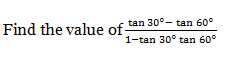a) 1/√3

b) – 1/√3

c) √3

d) – √3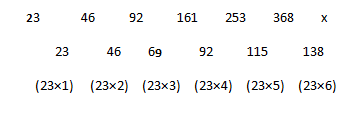M1D1 = M2D2

10M*12D = 8x

X = 15 Workers

15 – 12 = 3 more workers needed

(169 + 11) ÷6 (25)

180 ÷ 6 ×25

30 × 25 = 750

5935 + 5500 + 4290 = 15725

499 + 930 + 658 = 2087

15725÷2087 = 7.5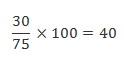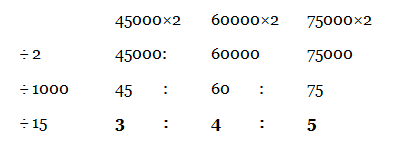16 – 4x = 16x + 4

16 – 4 = 16x + 4x

20x = 12

X = 3/5

S.I = PNR/100

132 = P * 2 * 5.5/100

P = (132 * 100)/(2*5.5 )= 13200 / 11 = 1200

Principal = 1200

Go by trial and error

Total distance cover by the boy = 42 km

He covered distance by car = 42* 2/7 = 12 km

Remaining Distance = 42 – 12 = 30 km

Distance covered by Bike = 30 *(1/3) = 10 km

Car + Bike = 12 + 10=22

42 – 22 = 20

Half of the distance of remaining= 10 km (Covered by bus)

Remaining Distance = 10 km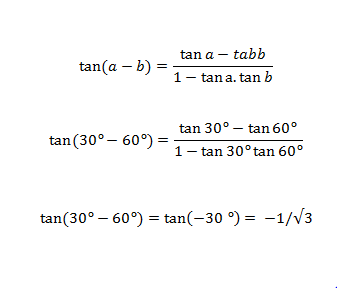RRB ALP 2018 – “All in One” Study Materials and Practice Sets